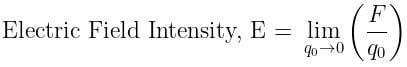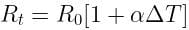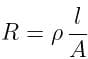Test: Electricity - 3

# Test: Electricity - 3 - Class 10

Test Description

## 25 Questions MCQ Test Science Class 10 - Test: Electricity - 3

Test: Electricity - 3 for Class 10 2023 is part of Science Class 10 preparation. The Test: Electricity - 3 questions and answers have been prepared according to the Class 10 exam syllabus.The Test: Electricity - 3 MCQs are made for Class 10 2023 Exam. Find important definitions, questions, notes, meanings, examples, exercises, MCQs and online tests for Test: Electricity - 3 below.
Solutions of Test: Electricity - 3 questions in English are available as part of our Science Class 10 for Class 10 & Test: Electricity - 3 solutions in Hindi for Science Class 10 course. Download more important topics, notes, lectures and mock test series for Class 10 Exam by signing up for free. Attempt Test: Electricity - 3 | 25 questions in 25 minutes | Mock test for Class 10 preparation | Free important questions MCQ to study Science Class 10 for Class 10 Exam | Download free PDF with solutions
 1 Crore+ students have signed up on EduRev. Have you?
Test: Electricity - 3 - Question 1

### The space around a charge in which its effect can be felt is called its

Detailed Solution for Test: Electricity - 3 - Question 1

The electric field is the region around a charged particle where the lines of force can be felt by another charge by getting repulsed or attracted as per their sign of charges.

Test: Electricity - 3 - Question 2

### The force acting on a unit positive test charge at a point inside an electric field is called

Detailed Solution for Test: Electricity - 3 - Question 2

Electric Field Intensity (E): The electrostatic force acting per unit positive charge on a point in an electric field is called electric field intensity at that point.Test: Electricity - 3 - Question 3

### Work done in moving a unit positive test charge from infinity to a point inside an electric field, is called

Detailed Solution for Test: Electricity - 3 - Question 3

Electric potential: An electric potential is defined as the amount of electric potential energy that a unitary point electric charge would have if located at any point of space.
It is equal to the work done by an electric field in carrying a unit positive charge from infinity to that point.

Test: Electricity - 3 - Question 4

Work done is moving a unit positive test charge from one point to other inside an electric field, is called

Detailed Solution for Test: Electricity - 3 - Question 4

Potential difference is the difference in electric potential energy between two points.
It is the amount of work done in moving a unit positive charge from one point to another point in an electric field. This is measured in volt.

Test: Electricity - 3 - Question 5

Electricity constituted by electric charges at rest on the surface of a conductor, is called

Detailed Solution for Test: Electricity - 3 - Question 5

Static electricity is an imbalance of electric charges within or on the surface of a material. The charge remains until it is able to move away by means of an electric current or electrical discharge.

Test: Electricity - 3 - Question 6

Electricity constituted by moving electric charges, is called

Detailed Solution for Test: Electricity - 3 - Question 6

Electricity constituted by moving electric charge is called current.

Test: Electricity - 3 - Question 7

Time rate of flow of electric charge measures electric _________.

Detailed Solution for Test: Electricity - 3 - Question 7

Current is defined as the amount of electric charge flowing through a cross-section of circuits in unit time.
I = Q/T

Test: Electricity - 3 - Question 8

The condition for an electric charge to flow from one point to other is that the two points must have electric

Detailed Solution for Test: Electricity - 3 - Question 8

As we know the current is the flow of charges so it requires a potential difference between the two points.

Test: Electricity - 3 - Question 9

The device that maintains electric potential difference between two points is called electric

Detailed Solution for Test: Electricity - 3 - Question 9

Cell is the device which is used to maintain the potential difference between two points of the conductor.

Test: Electricity - 3 - Question 10

The path connecting two points at different potentials, to make the electric charges flow, is called electric _________.

Detailed Solution for Test: Electricity - 3 - Question 10

An electric circuit is the representation of the whole path of current and also the various devices connected in it, like Cell, Resistor, etc.

Test: Electricity - 3 - Question 11

The device which measures electric potential difference between two points is called

Detailed Solution for Test: Electricity - 3 - Question 11

A voltmeter is an instrument used for measuring the electrical potential difference between two points in an electric circuit.

Test: Electricity - 3 - Question 12

The device which measures electric current through a conductor is called

Detailed Solution for Test: Electricity - 3 - Question 12

Ammeter is used to measure electric current because it has very low resistance and are always connected in series

Test: Electricity - 3 - Question 13

Electric current is produced by flow of

Detailed Solution for Test: Electricity - 3 - Question 13

An electric current is said to exist when there is a net flow of electric charge through a region. In electric circuits this charge is often carried by electrons moving through a wire.

Test: Electricity - 3 - Question 14

Direction of flow of conventional current is taken from

Detailed Solution for Test: Electricity - 3 - Question 14

Conventional current or simply current, behaves as if positive charge carriers cause current to flow. Conventional current flows from the positive terminal to the negative.

Test: Electricity - 3 - Question 15

The law which gives a relation between electric potential difference and electric current is called

Detailed Solution for Test: Electricity - 3 - Question 15

Ohm's law states that the current through a conductor between two points is directly proportional to the voltage across the two points.

Test: Electricity - 3 - Question 16

With increase in temperature, resistance of a conductor

Detailed Solution for Test: Electricity - 3 - Question 16

As the temperature of a metallic conductor increases, the kinetic energy of the electrons of the conductor also increases
Due to this increase in kinetic energy more obstruction is offered to the flowing electrons i.e. current.
Therefore, as more obstruction is offered to the flowing electrons, the resistance is increased.Test: Electricity - 3 - Question 17

In series combination, total resistance

Detailed Solution for Test: Electricity - 3 - Question 17

When two or more resistors are connected in series, the total resistance of the combination is equal to the sum of all the individual resistances.

Thus, the total resistance of the circuit will always increase.

Test: Electricity - 3 - Question 18

In parallel combination, total resistance

Detailed Solution for Test: Electricity - 3 - Question 18

To find the total resistance of all components, the reciprocals of the resistances of each component are added and the reciprocal of the sum is taken.

Thus, the Total resistance will always be less than the value of the smallest resistance.

Test: Electricity - 3 - Question 19

In series combination, resistance increases due to increase in

Detailed Solution for Test: Electricity - 3 - Question 19where,
R = resistance,
ρ = resistivity,
ℓ = length,
A = area of cross-section

From the above relation: R ∝ ℓ
⇒ resistance increases due to an increase in length
Because the electrons have suffered greater collisions with atoms in the material.

Test: Electricity - 3 - Question 20

In parallel combination, resistance decreases due to increase in

Detailed Solution for Test: Electricity - 3 - Question 20

In parallel combination, change in length will not be effective, therefore we consider the area of cross-section.
As we know that resistance is inversely proportional to the area of cross-section.

Thus, resistance will decrease with an increase in area of crosssection.

Test: Electricity - 3 - Question 21

When electric current is passed, electrons move from

Detailed Solution for Test: Electricity - 3 - Question 21

Since electrons are negative they should be repelled by the negative charge and attracted by the positive charge, i.e., in an electric field the electrons must move from regions of low potential to the regions with high potential by the action of the electrical forces.

Test: Electricity - 3 - Question 22

Electrical resistivity of any given metallic wire depends upon

Detailed Solution for Test: Electricity - 3 - Question 22

Electrical resistivity of a given metallic wire depends on number density of free electrons in the conductor which is the nature of material

Test: Electricity - 3 - Question 23

In an atom, particle having a positive charge is called

Detailed Solution for Test: Electricity - 3 - Question 23

A positively charged subatomic particle that is housed within the nucleus is caled Proton.

Test: Electricity - 3 - Question 24

In an atom, particle having a negative charge, is called

Detailed Solution for Test: Electricity - 3 - Question 24

A negatively charged subatomic particle that has negligible mass and moves around in a cloud around the nucleus is called Electron.

Test: Electricity - 3 - Question 25

Substances whose atoms have more free electrons, are called

Detailed Solution for Test: Electricity - 3 - Question 25

Materials with high electron mobility (many free electrons) are called conductors, while materials with low electron mobility (few or no free electrons) are called insulators.

## Science Class 10

78 videos|509 docs|153 tests
Information about Test: Electricity - 3 Page
In this test you can find the Exam questions for Test: Electricity - 3 solved & explained in the simplest way possible. Besides giving Questions and answers for Test: Electricity - 3, EduRev gives you an ample number of Online tests for practice

## Science Class 10

78 videos|509 docs|153 tests